Get instant live expert help with Excel or Google Sheets“My Excelchat expert helped me in less than 20 minutes, saving me what would have been 5 hours of work!”

#### Post your problem and you’ll get expert help in seconds.

Your message must be at least 40 characters
Our professional experts are available now. Your privacy is guaranteed.

# How to Automatically Sort in Excel – Excelchat

We can do Automatic Sorting in Excel using a very simple method. This tutorial will guide all levels of Excel users on how to do automatic sorting in excel.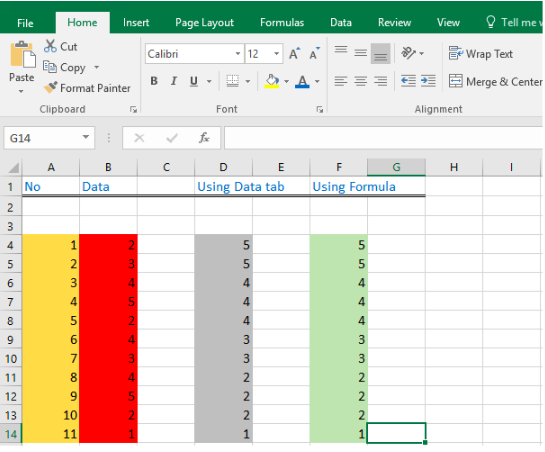Figure 1: Automatic Sorting in excel

## Automatic Sorting in Excel

• We will select the data, copy it and paste it in another Column D.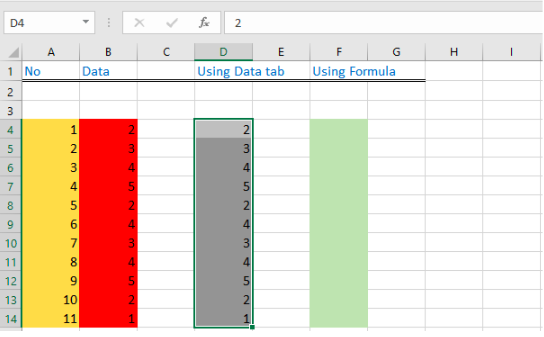Figure 2: Data table

• We will click on Data tab and then we will click on A-Z tab to sort the data manually in ascending order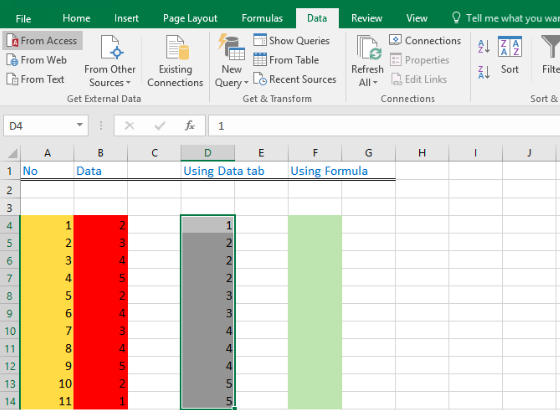Figure 3: Highlighted sorted data in ascending order

• We will click on Z-A tab to sort the data manually in descending order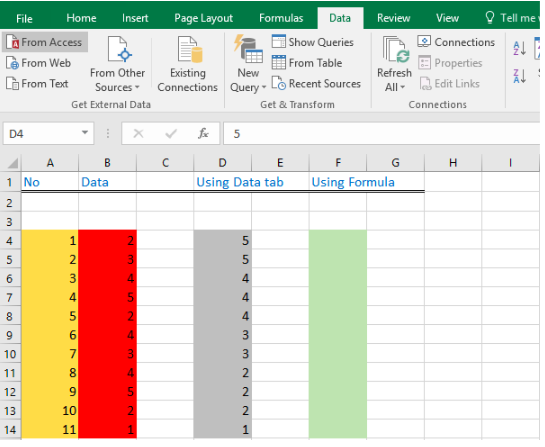Figure 4: Highlighted sorted data in descending order

## Auto sort with Formula in Excel

• To auto sort with formula, we can use the formula `=LARGE(B4:B14)`.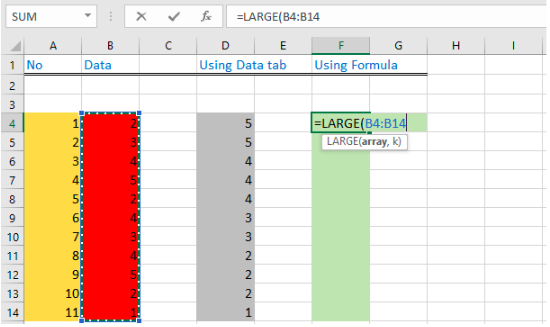Figure 5: Excel auto sort table

• We will insert the dollar sign so that the selection does not change as we move the formula from Cell B4 to Cell B5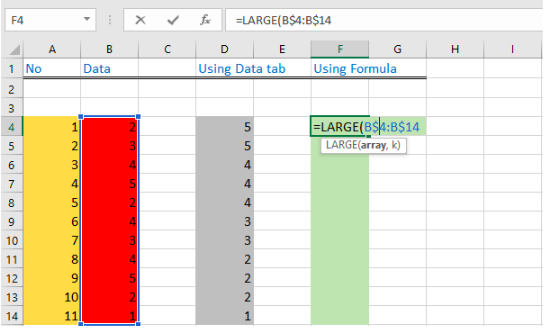Figure 6: Inserted dollar sign to formula

• We will insert the first largest number A4 in the formula.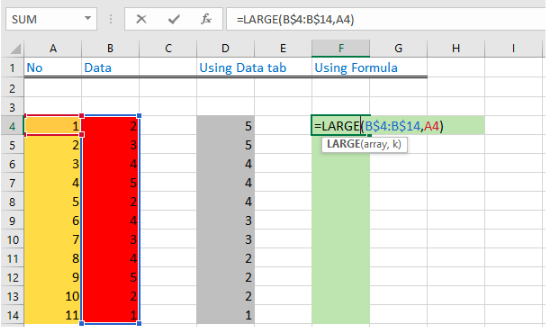Figure 7: Inserted first largest number to the formula

• We will then click OK button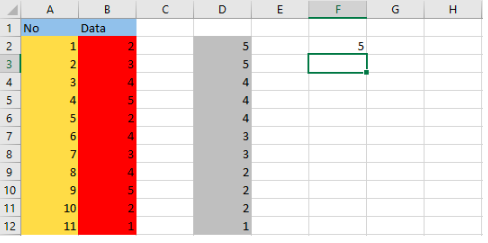Figure 8: Automatic sort first large number to table

• We will drag the cell down to get the second largest number A5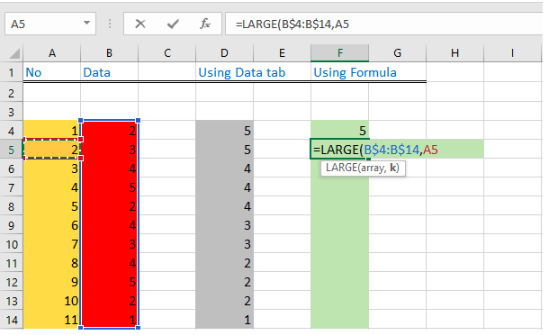Figure 9: Inserted second largest number to the formula

• We will drag down to F14 and then click on the OK button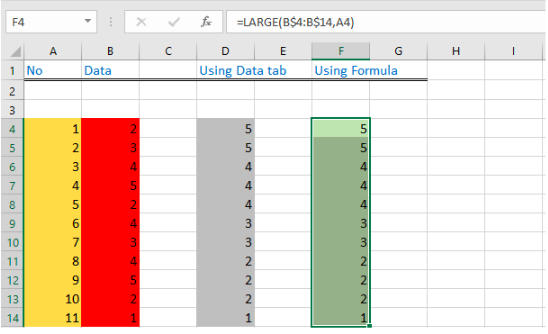Figure 10: Inserted excel auto sort table

• This way we can sort the number without clicking on the Data tab and then A-Z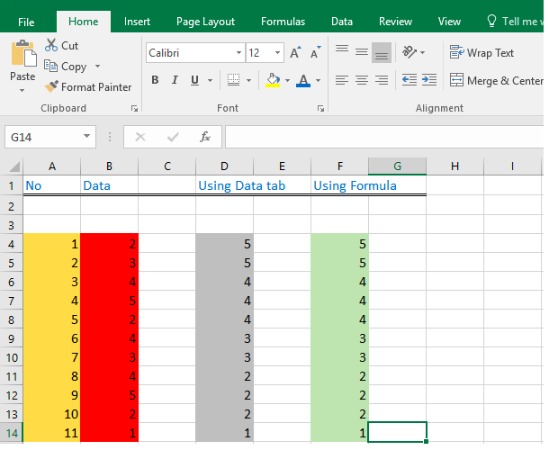Figure 11:   Excel Auto Sort Table

## How to Auto sort in Excel when Data Changes

• To auto sort in excel when data changes, the sorting we did using Data tab will not change automatically but the sorting using Formula will change.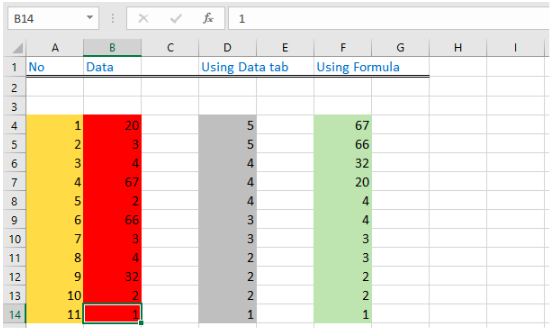Figure 12: Excel auto sort when data changes

• We will find it much easier to sort with excel using formula than using the data tab.

## Instant Connection to an Expert through our Excelchat Service

Most of the time, the problem you will need to solve will be more complex than a simple application of a formula or function. If you want to save hours of research and frustration, try our live Excelchat service! Our Excel Experts are available 24/7 to answer any Excel question you may have. We guarantee a connection within 30 seconds and a customized solution within 20 minutes.